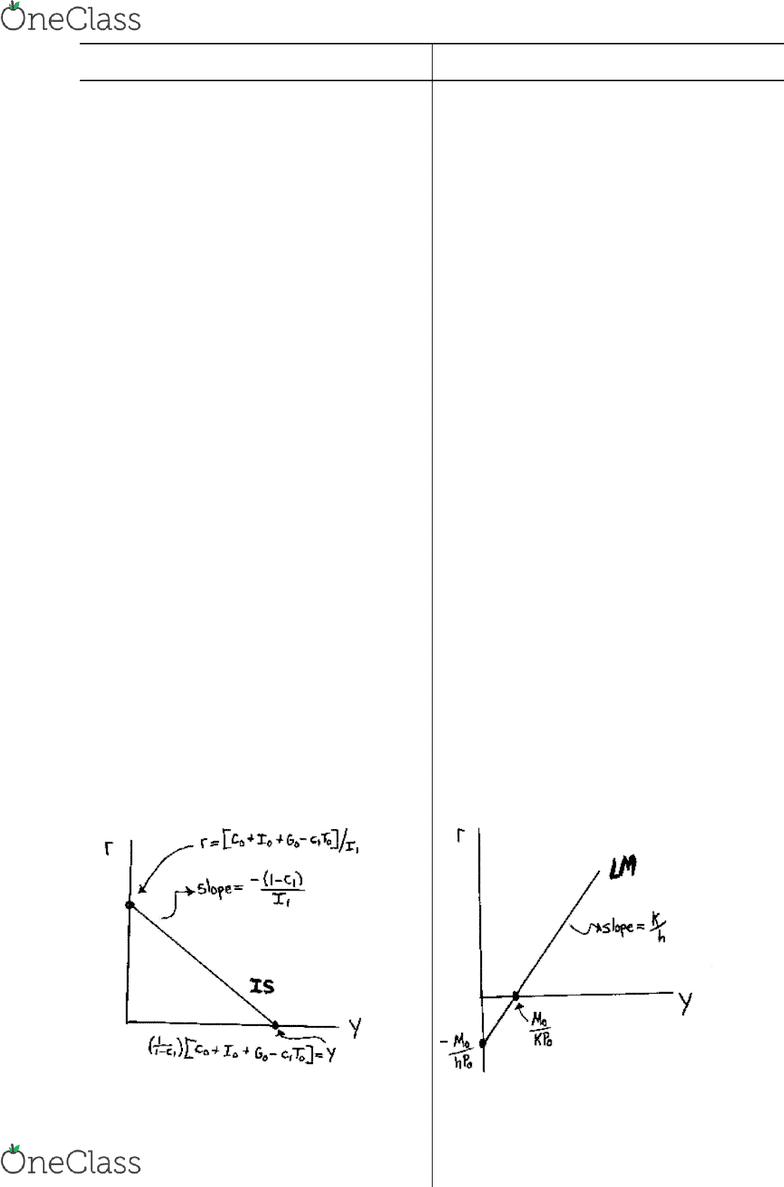Class Notes (1,100,000)
CA (640,000)
UTSC (30,000)
Iris Au (30)
Lecture 8

# MGEB06H3 Lecture Notes - Lecture 8: Ceteris Paribus, Real Interest Rate, Keynesian Cross

Department
Economics for Management Studies
Course Code
MGEB06H3
Professor
Iris Au
Lecture
8

This preview shows pages 1-2. to view the full 6 pages of the document.1
Review of IS/LM Model (Chapters 10 & 11)
IS Curve
LM Curve
Ceteris paribus, the Investment Saving or IS
Curve shows all of the (Y,r) pairs that
support (lead to) equilibrium in the Goods and
Services market.
( i.e. YS = YD S = I )
Ceteris paribus, the Liquidity Market or LM
Curve shows all of the (Y,r) pairs that
support (lead to) equilibrium in the Money
(Asset) market.
( i.e. MS = MD AssetD = AssetS )
Keynesian Cross
Short-Run (SR) Equilibrium output (Y*SR)
is determined by equating planned
expenditure (E = YD) to actual expenditure
(Y = YSSR) GIVEN among other things fiscal
policy (i.e. G & T) and the real interest rate
(thus I is fixed).
Theory of Liquidity Preference
The real interest rate varies to determine
equilibrium in the money/assets market
GIVEN output (Y); the money supply (MS);
the aggregate or average price level (P); and
the real money demand curve L(Y,r).
In essence, the IS Curve combines the
interaction between r and I expressed by the
investment function and the interaction
between I and Y demonstrated by the
Keynesian cross.
Having r rise (fall) shifts E down (up) thereby
decreasing (increasing) Y*SR.. This traces out
the IS Curve as downward sloping.
Money market equilibrium implies all asset
markets are in equilibrium. Walras’ Law
states that if all but one market is in
equilibrium then the last market is also.
Therefore, consider assets as consisting of
two types monetary (money) and non-
monetary assets then MS = MD AssetD =
AssetS.
Having Y rise (fall) shifts the real money
demand function ( L(Y,r) ) up (down) thereby
increasing (decreasing) r*SR. This traces out
the LM Curve as upward sloping.
find more resources at oneclass.com
find more resources at oneclass.com

Only pages 1-2 are available for preview. Some parts have been intentionally blurred.2
IS Curve
LM Curve
Algebraic Solution
NX = 0 Closed economy
C = C0 + C1•( Y - T ) Consumption Function
I = I0 - I1r Investment Function
G = G0 Exogenous FP
(spending policy)
T = T0 Exogenous FP (tax
policy)
Y = C + I + G NII
IS Curve
Y = [ 1/(1-C1) ][ C0 + I0 + G0 - C1T0 ]
- ( I1/(1-C1) )r
derived/drawn for: C0, I0, G0, T0, C1 & I1
given, fixed or exogenous
Y-axis intercept:
Y = [ 1/(1-C1) ][ C0 + I0 + G0 - C1T0 ]
r-axis intercept:
r = [ C0 + I0 + G0 - C1T0 ]/I1
IS Slope: δr/δY = - (1-C1)/I1 < 0
Algebraic Solution
Md = P( kY - hr ) Nom money
demand
Md/P = k•r - hr Real money
demand
MS = M0 Exogenous MP (exog.
nominal money supply)
P = P0 Exogenous or fixed P level
MS = Md or MS/P = Md/P
LM Curve
Y = (1/k)(M0/P0) + ( h/k )r
derived/drawn for: M0S, P0, k & h given,
fixed or exogenous
Y-axis intercept:
Y = (1/k)(M0/P0)
r-axis intercept:
r = ( -1/h )(M0/P0)
LM Slope: δr/δY = k/h > 0
find more resources at oneclass.com
find more resources at oneclass.com